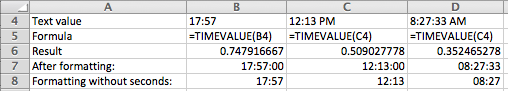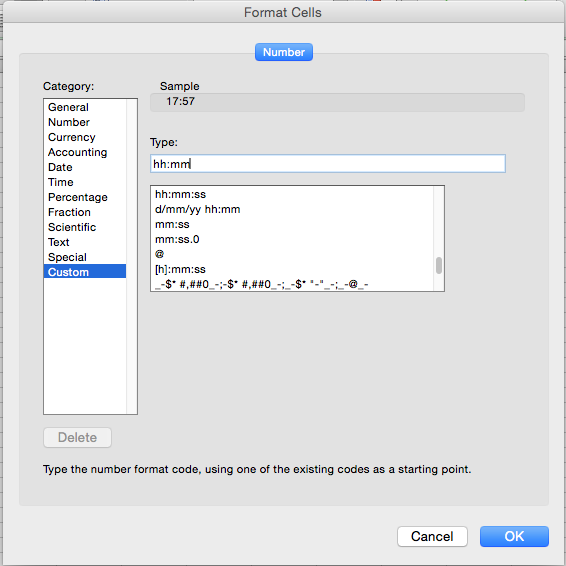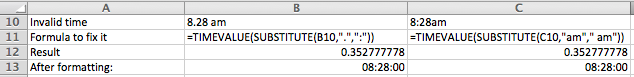# Use the TIMEVALUE function to convert text to time in Excel

If you have a spreadsheet with time values that have been added to the spreadsheet as text values, you need the TIMEVALUE function. This will allow you to convert the text values into valid time values.

## TIMEVALUE() Syntax

The TIMEVALUE function is used to convert a text value into a time value. It has the following syntax:

=TIMEVALUE(time_text)

## The TEXTVALUE Function - worked example

To use this function, you need a cell that contains a text value which has been entered to look like a time. An example might be "12:13 PM", "17:57" or "8:27:33 AM". You are most likely to encounter this in a spreadsheet that has been imported from another format, such as a CSV or text file. If you try typing values like this directly into Excel, you'll find that Excel recognizes them as time values and automatically converts them for you.

In the following screenshot, you'll see the TIMEVALUE function in action for the two examples given above:Note that row 6 contains the actual result of the TIMEVALUE formula. When Excel converts the text to a time value, it doesn't automatically apply a format to the result - it simply stores the number that your time value represents.

What you're seeing in the Result row is the time in B4 expressed as a fraction of 1 day. For example, if you multiply the value in B4 by 24, you'll get 17.95, i.e. 17.95 hours out of a total of 24.

## Formatting the Date Result

In many scenarios, you'll want to format the result of your TIMEVALUE formula so it displays as an actual time. Rows 7 and 8 show a couple of examples of how this number can be formatted to suit your needs.

Row 7 has the default Time format applied from the Number format list in the ribbon toolbar. This gives us the time value expressed in hours, minutes and seconds (hh:mm:ss).

Row 8 uses a custom time format that excludes the seconds value from the display:

• Choose Custom from the Number format list• You can then specify the exact format you want as shown here:\
• As you can see, the Custom list contains a number of different custom time format options (as well as other custom formats). I didn't see the one I wanted, so I simply edited an existing format using hh:mm.
• It is very important to note that applying a time format to a number does not change the number itself. This matters if you decide to perform any calculations using the time values.

## Troubleshooting the TIMEVALUE function

As noted earlier, the TIMEVALUE function only works on text values that look like standard Excel formats.

In some cases, your TIMEVALUE formulas will return a #VALUE result, indicating that Excel didn't recognise the text as a valid value. This can be frustrating, especially if you can't see any problems with the values you are supplying.

Here are two examples of time values that Excel doesn't recognise:

• 8.28 am (Excel doesn't like the period/point between the 8 and 2.
• 8:28am (Excel doesn't like the fact there is no space before "am")

Another reason your TIMEVALUE formula might return a #VALUE error is that Excel is already storing the value as a time value. Try applying a different time format to the cell to see if this is the case.

If you are having a problem with a specific time format that isn't on this list, please let us know through the comments so we can solve it for you, and add it to this list. You could also read our lesson on converting text into dates, which has some additional troubleshooting tricks you could try.

To solve these specific examples, you can use the SUBSTITUTE function as part of your TIMEVALUE formulas to successfully convert the text into time values. The SUBSTITUTE function takes all instances of a text string and replaces it with another as shown here:As you can see, the formula in B11 replaces the point with a colon, which fixes the text in B10 so it can be converted successfully.

The formula in C11 is a bit more tricky. It uses the SUBSTITUTE function to replace "am" with " am" (note the addition of the space in front of am), so Excel will recognise the text as a valid value.

## Summary

In this lesson, we looked at how the TIMEVALUE function can be used to convert a text value into a time value. It requires that the text value look like a valid time that Excel will recognise. We looked as some examples of text values that Excel recognised, and then identified some that Excel doesn't - and how to fix them.

If you have any questions or suggestions regarding this lesson, feel free to use the comments section below to let us know.

## Our Comment Policy.

Add a comment to this lesson

### Try this

Instead of all that, try highlighting the whole column. Then click on Text to Columns. Sounds odd, i know. Then just click Finish. It magically converts time or dates as a normal time or date...versus text.

### Converting hh.mm to hh:mm

Your information about how to convert hh.mm to hh:mm was very helpful, but when I try to convert to 9.00 to hh:mm it doesn't works. Excel return an #VALUE!. Any recommendation?

### =TIMEVALUE(SUBSTITUTE(A1,"AM"," AM")).

Thank you for your information above. Can I have one formula that address no space before "am" AND "pm", in the same formula.

### Trouble converting text to time format

Hi so I have this set of data extracted from a system, the time appears as this: 09:27:20

I have tried to use the =timevalue function to convert it but failed, the results come out as #value. I don't know what is issue. Are you able to help please?

### Check that it isn't already stored as a time value

Hi Hayley

The TIMEVALUE function turns a text string into a time. Because it's returning #VALUE, this suggests that the data is already a time value. The easiest way to test this is to apply a different format to it and see what happens.

I pasted your example into Excel and tried applying a couple of different formats to the cell.

• When I cleared the formatting, the value in the cell changed to 0.393981481
• I then applied a different time format to the cell, and it then appeared as 9:27:20 AM

In my case, this indicates that Excel treated the value I pasted as a time value, so the TIMEVALUE function won't work. You should check the values you're trying to convert to see whether that is the case in your spreadsheet as well.

### convert text intended as time to time

Need to convert time, showing as text, entered as hours, but without the symbol ":" to time. Example converting 1300 to 13:00 (or 1 pm), 1600 to 16:00 or (4:00 pm). Aim is to assess how many events occurred between time X and time Y. Example, how many customers entered the store between 1300 and 1600. Thanks for your help!!

### Date Format/Convert in Excel 2016

I wanted to convert 30/10/2017 2:48:11 PM to DD/MM/YYYY HH:MM (Time in 24 Hours format). Please help me. TIMEVALUE formula returning a #VALUE error.

### convert dtring of numbers to time

I have a report that shows the last time a journal entry was updated. I want to format that information for cut-off purposes. for example, I want to isolate any journal entries posted after noon on any given day. My update time shows as 9330400, and time formats I try to apply either result in "#######" or #Value!

### 7.2 displays as 07:02 not 07:20

I have followed the above lesson to convert numbers such as 7.05, 7.10, 7.15 into times 07:05, 07:10 and 07:15, but when it comes to the 7.10 it displays as 07:01 rather than 07:10 that I would expect. This is the same for all the round numbers - 7.2 is 07.02 not 07.20. Is there a way to ensure these are converted identically? I have used =TIMEVALUE(SUBSTITUTE(A1,".",":")) - it obviously also fails for values on the hour when recorded just as 7. It produces #VALUE!
What can I do?
Thanks

### Time that Contains Works

Hello, I'm looking for an easy way to convert time from text format to time in minutes. For example, the current cell shows [ 5 hr 47 min ] and I would like to use a formula to covert it to HH:MM:SS [ 5:47:00 ] OR MM:SS [ 347:00 ]. I have 1,300+ results to convert so if I can learn of an easier way, that would be great!

Marisol

### The solution I came up with

The solution I came up with was to first parse the data into individual text time components, then use the =TIME(hour, minute, second) function to convert the text components to time.

The following is a working example of the solution using your time text data in HH:MM:SS format:

A1: 5:47:00 (formatted as Text; 5 hours, 47 minutes, 00 seconds)
B1: =LEFT(A1,FIND(":",A1)-1)
C1: =MID(A1,FIND(":",A1)+1,FIND(":",A1,FIND(":",A1)))
D1: =MID(A1,FIND(":",A1,FIND(":",A1)+1)+1,2)
E1: =TIME(B1,C1,D1) (formatted as Custom: HH:MM:SS)

Copying the formulas in columns B:E to each row you have data in A column for, you can then: =SUM(E1:Ex), where x = the last row of your data, and you will get the total number of hours, minutes, and seconds in the list.